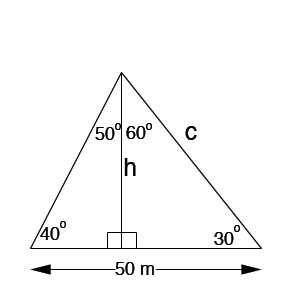SEARCH HOMEMath Central Quandaries & QueriesHeidi, a student: find the height of a triangle, which can be split into two right triangles, but the base (50m) is not split equally in half. one end of the base is 40 degrees, while the other is 30 degrees.Hi Heidi,

After finding all of the angles of the triangle:Use the law of sine to solve for either other side, using the 110o and the side of 50 m. For example:

(sin 110)/50=(sin 40)/c

Once you know the length of the sides of a right triangle, you can now use the appropriate primary trig function -- sine, cosine or tangent.

Hope this helps,

JaniceMath Central is supported by the University of Regina and The Pacific Institute for the Mathematical Sciences.